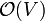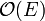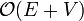# Difference between revisions of "Breadth-first search"

Breadth-first search (BFS) is the type of graph search that arises when the oldest vertex on the fringe is always the first to leave it. Though its uses are not as widespread as those of DFS, its specific application to the problem of finding shortest paths in unweighted graphs provides an example of a model algorithm: an algorithm which solves one particular, precisely specified (yet important) problem, solves it well (in minimal time and space), and is easy to understand and to implement.

## Implementation

The basic template given at Graph search can be easily adapted for BFS:

input G
for all u ∈ V(G)
let visited[u] = false
let Q be empty
let r be a node of depth zero
push(Q,r)
while Q is not empty
v = pop(Q)
if visited[v] = false
visit v
visited[v] = true
for each w ∈ V(G) such that (v,w) ∈ E(G) and visited[w] = false
push(Q,w)


F has been replaced by a queue, Q, because a queue satisfies exactly the necessary property for BFS: the most recently added item is the last to be removed.

## Performance characteristics

### Time

Most BFS algorithms feature a constant time "visit" operation. Suppose, for the sake of simplicity, that the entire graph consists of one connected component. Each vertex will be visited exactly once, with each visit being a constant-time operation, so$\mathcal{O}(V)$ time is spent visiting vertices. Whenever a vertex is visited, every edge radiating from that vertex is considered, with constant time spent on each edge. It follows that every edge in the graph will be considered exactly twice — once for each vertex upon which it is incident. Thus, the time spent considering edges is again a constant factor times the number of edges,$\mathcal{O}(E)$. This makes BFS$\mathcal{O}(E+V)$ overall, linear time, which, like DFS, is asymptotically optimal for a graph search.

(The foregoing analysis is not rigorous, because it is possible to have multiple copies of a vertex on the queue at any given time. However, we can easily fix this by adding a boolean array to prevent pushing a vertex onto the queue multiple times, and even if we don't, a more detailed analysis shows that the time used is still linear.)

### Space

There can be no more than$\mathcal{O}(E)$ vertices on the queue at any given time, because every addition of a vertex to the stack is due to the traversal of an edge (except for that of the initial vertex) and no edge can be traversed more than twice. The space required by the queue is therefore bounded by$\mathcal{O}(E)$.$\mathcal{O}(V)$ space is also required to store the list of visited vertices, so$\mathcal{O}(E+V)$ space is required overall.

## Applications

BFS, by virtue of being a subcategory of graph search, is able to find connected components, paths (including augmenting paths), spanning trees, and topological orderings in linear time. In addition, BFS is the algorithm of choice for finding shortest paths in unweighted graphs.

The following example shows how to find connected components and a spanning forest (a collection of spanning trees, one for each connected component) with BFS.

input G
let cnt = 0
for all u ∈ V(G)
let id[u] = -1
for each u ∈ V(G)
if id[u] = -1
let Q be empty
push(Q,(u,u))
while Q is not empty
(u,v) = pop(Q)
if id[v] = -1
id[v] = cnt
let pred[v] = u
for each w ∈ V(G) such that (v,w) ∈ E(G)
push(Q,(v,w))
cnt = cnt + 1


After the termination of this algorithm, cnt will contain the total number of connected components, id[u] will contain an ID number indicating the connected component to which vertex u belongs (an integer in the range [0,cnt)) and pred[u] will contain the parent vertex of u in some spanning tree of the connected component to which u belongs, unless u is the first vertex visited in its component, in which case pred[u] will be u itself.

The spanning trees produced by BFS tend to be broad, with high branching factor, unlike those produced by DFS, which tend to be tall and thin.